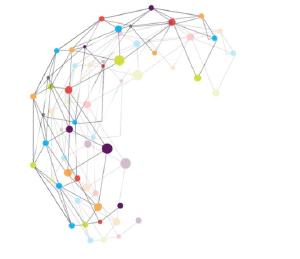# ARC: research project "Algebra"

From algebra to combinatorics, and backIn this project, researchers will study several related questions in algebra and geometry, by merging of combinatorial and categorical techniques.

In algebraic geometry, one studies so-called "algebraic spaces", which are geometric objects that are fully described by their associated “coordiante algebra”. The geometric properties of the considererd space can henceforth be translated into algebraic properties of the associated algebra.

The advantage of this approach is, that in this algebraic setting, a whole new range of tools becomes available that are not at hand in the purely geometric setting.
For example, if the initial space contains “singularities” (that is, points where tangent spaces no longer exist in the classical sense), it can be useful to consider “non-commutative” algebras, which are algebras that do not arise as coordinate algebras from geometric objects in the classical sense, but which allow to “resolve” these singularities in a way that can be precisely defined. The first part of this project concerns such “non-commutative (crepant) resolutions”.

Subsuming the above mentioned correspondance between “algebraic spaces” and “commutative algebras”, to any (possibly non-commutative) algebra, we can associate its “representation category” and interpret this as a geometric object.

In this project researchers aim to go a step further and study categories of “partial representations”, in particular of finite and algebraic groups. As groups themselves arise naturally in geometry as they describe symmetries, partial actions and representations describe in a similar way symetries that are not globally defined, which allows us to probe deeper the internal structure of the considered geometric object, such as as a polytope.

Coordinator : Joost Vercruysse, Département de mathématique, Faculté des Sciences
Partners : Michele D’Adderio, Dimitri Leemans, Spela Spenko

Dates
Created on September 3, 2020